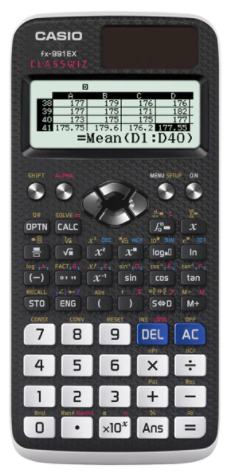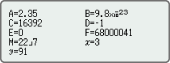# Casio FX-991EX Scientific Calculator #C63*Image may differ with actual product's layout, color, size & dimension. No claim will be accepted for image mismatch.

# Casio FX-991EX Scientific Calculator #C63

Product Id: 130.01.685.51

Regular Price  1,630
Special Price  1,550

Delivery time?

Quick Overview

Model - Casio FX-991EX
Brand - Casio
Type - Scientific Calculator
Digit - 12 digits (10 + 2 digits)
Display - Dot matrix display
Key - Plastic keys
Model
Casio FX-991EX
Type
Scientific Calculator
Display
Dot matrix display
Digit
12 digits (10 + 2 digits)
Key
Plastic keys
Number of Functions
552
Power Sources
Solar & Battery
Dimensions
11.1 x 77 x 165.5mm
Weight (Kg)
90gm
Specialty
GCSE/AS/A Level, Suitable for Cambridge International Examinations GCSE, AS & A Level, Natural textbook display, Display expression same as textbook, Solar & Battery, Solar powered when light is sufficient, battery powered when light is insufficient, Intuitive, easy-to-learn icon display, List display function for thorough, speedy learning, Easy-to-understand English messages and interactive format, High computational competence for performing even advanced mathematics, Online Visualization Service Using QR Code, A comprehensive set of advanced functions unique to CASIO scientific calculators, 10 + 2 digits, Dot matrix display, Icon menu, Multi-replay, Plastic keys, Metric conversion function, Ratio, Basic Mathematical Functions, Table Generation, Intermediate Statistics, Base-n calculation, Scientific Constants, Vector, Advanced Statistics, Inequality, List based STAT-data editor, Differentiation, Spreadsheet, Non Graphing, Matrix, Equation, Integration, High Resolution, Complex number calculation, QR Code, Basic Statistics
Others
Part no: C63, GCSE/AS/A Level: Suitable for Cambridge International Examinations GCSE, AS & A Level, Natural textbook display: Display expression same as textbook, 10 + 2 digits: 10-digit mantissa + 2-digit exponential display, Dot matrix display: High-resolution screen provides beautiful looking graphs every time, Icon menu: Specify the operation you want to perform by selecting an icon or inputting a number, Multi-replay: Quick and easy recall of previously executed formulas for editing and re-execution, Solar & Battery: Solar powered when light is sufficient, battery powered when light is insufficient, Plastic keys: Designed and engineered for easy operation, Metric conversion function: can convert a number in one unit to a number in a different unit, Basic Mathematical Functions: Trigonometric, Exponential logarithmic, etc., Table Generation: can create a numerical table based on that expression by registering a function expression. In addition, graph functions can be represented by graphs, Intermediate Statistics: More statistical functions such as Paired-variable statistical calculation, Quartiles and List display functions for speedy and thorough learning, Base-n calculation: Binary/Octal/Hexadecimal, Scientific Constants: can use constant symbols used in physics, science classes, Advanced Statistics: Support high level statistics by 7 kinds of advanced distribution calculation, List based STAT-data editor: Viewing and editing of input data in list format, showing data groups (x-data, y-data, frequency) and surrounding data, High Resolution: choosing a model with higher definition in our non-programmable model and a higher definition model with Programmable model, QR Code: can see graphs on smartphones etc. using QR Code, Basic Statistics: Standard statistics functions such as Mean, SUM, Standard Deviation, and Regression
Warranty
3 year

## fx-991EX### Intuitive, easy-to-learn icon displayThe use of icons on the menu screen improves viewability. Selection of the desired function is quick and easy.

### Natural Textbook DisplayInput and display fractions, powers, logarithms, roots, and other mathematical formulas and symbols just as they appear in textbooks.

### List display function for thorough, speedy learningVariables and statistical calculation results stored in memory can be displayed in lists. There’s no need to recall and confirm individual values as with previous models.

### Easy-to-understand English messages and interactive formatNearly all English words are displayed on the screen in unabbreviated form. Interactive menu display realizes more intuitive operation.

### High computational competence for performing even advanced mathematicsClassWiz contains calculation functions that support even advanced mathematical operations, including spreadsheet calculations, 4 × 4 matrix calculations, calculation of simultaneous equations with four unknowns and quartic equations, and advanced statistical distribution calculations.

### Online Visualization Service Using QR CodeGenerate QR codes of equations input into the calculator by a simple operation. Graphs and other graphics can be displayed on smartphone or tablet screens.

### A comprehensive set of advanced functions unique to CASIO scientific calculators

Spreadsheet calculationsUseful for learning statistics. Up to 5 columns × 45 rows (maximum of 170 data items)

Matrix calculationsPerform calculations with matrices of up to 4 rows and 4 columns.

Vector calculationsPerform calculations using up to four third-order vectors stored in memory.

Multiline displayFormulas are automatically wrapped to multiple lines. *

*In linear input mode

Integration calculationsPerform integration calculations in advanced mathematics.

Differential calculations>

Perform differential calculations in advanced mathematics.

Equation calculationsCalculation of simultaneous equations in 2 to 4 unknowns and high-degree equations of second to fourth degree

Inequality calculationsSolve second-degree to fourth-degree inequalities.

Advanced statistical distribution calculationsPerform calculations involving normal distributions, binomial distributions, and Poisson distributions.

Ratio calculationsPerform ratio calculations such as A:B=X:D.

Metric conversionsSelect units from the Metric Conversion Command List.

Scientific constantsSelect scientific constants from the Scientific Constant Table.

Variables listOne-touch list display

Statistics listDisplays six rows at once.

Digit separatorSeparation every three digits makes even large numbers easy to read.

Engineering symbolsPerform engineering calculations that include symbols such as k, M, m, and n.

Model - Casio FX-991EX, Brand - Casio, Type - Scientific Calculator, Digit - 12 digits (10 + 2 digits), Display - Dot matrix display, Key - Plastic keys, Number of Functions - 552, Power source - Solar & Battery, Dimension - 11.1 x 77 x 165.5mm, Weight - 90gm, Others - Part no: C63, GCSE/AS/A Level: Suitable for Cambridge International Examinations GCSE, AS & A Level, Natural textbook display: Display expression same as textbook, 10 + 2 digits: 10-digit mantissa + 2-digit exponential display, Dot matrix display: High-resolution screen provides beautiful looking graphs every time, Icon menu: Specify the operation you want to perform by selecting an icon or inputting a number, Multi-replay: Quick and easy recall of previously executed formulas for editing and re-execution, Solar & Battery: Solar powered when light is sufficient, battery powered when light is insufficient, Plastic keys: Designed and engineered for easy operation, Metric conversion function: can convert a number in one unit to a number in a different unit, Basic Mathematical Functions: Trigonometric, Exponential logarithmic, etc., Table Generation: can create a numerical table based on that expression by registering a function expression. In addition, graph functions can be represented by graphs, Intermediate Statistics: More statistical functions such as Paired-variable statistical calculation, Quartiles and List display functions for speedy and thorough learning, Base-n calculation: Binary/Octal/Hexadecimal, Scientific Constants: can use constant symbols used in physics, science classes, Advanced Statistics: Support high level statistics by 7 kinds of advanced distribution calculation, List based STAT-data editor: Viewing and editing of input data in list format, showing data groups (x-data, y-data, frequency) and surrounding data, High Resolution: choosing a model with higher definition in our non-programmable model and a higher definition model with Programmable model, QR Code: can see graphs on smartphones etc. using QR Code, Basic Statistics: Standard statistics functions such as Mean, SUM, Standard Deviation, and Regression, Specialty - GCSE/AS/A Level, Suitable for Cambridge International Examinations GCSE, AS & A Level, Natural textbook display, Display expression same as textbook, Solar & Battery, Solar powered when light is sufficient, battery powered when light is insufficient, Intuitive, easy-to-learn icon display, List display function for thorough, speedy learning, Easy-to-understand English messages and interactive format, High computational competence for performing even advanced mathematics, Online Visualization Service Using QR Code, A comprehensive set of advanced functions unique to CASIO scientific calculators, 10 + 2 digits, Dot matrix display, Icon menu, Multi-replay, Plastic keys, Metric conversion function, Ratio, Basic Mathematical Functions, Table Generation, Intermediate Statistics, Base-n calculation, Scientific Constants, Vector, Advanced Statistics, Inequality, List based STAT-data editor, Differentiation, Spreadsheet, Non Graphing, Matrix, Equation, Integration, High Resolution, Complex number calculation, QR Code, Basic Statistics, Warranty - 3 year

## Your review## Your Question